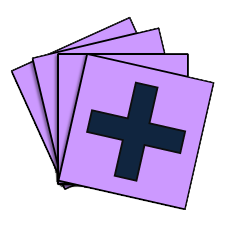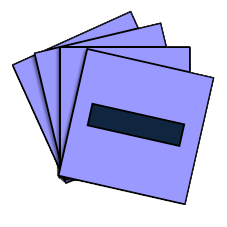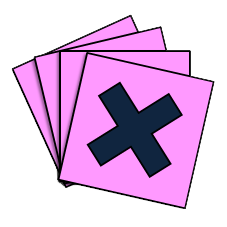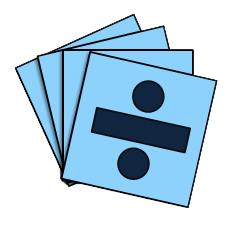You may also likePebbles

Place four pebbles on the sand in the form of a square. Keep adding as few pebbles as necessary to double the area. How many extra pebbles are added each time?Bracelets

Investigate the different shaped bracelets you could make from 18 different spherical beads. How do they compare if you use 24 beads?Sweets in a Box

How many different shaped boxes can you design for 36 sweets in one layer? Can you arrange the sweets so that no sweets of the same colour are next to each other in any direction?

Make 100

Make 100

Find at least one way to put in some operations signs ($+$, $-$, $\times$, $\div$) to make these digits come to $100$.

1    2    3    4    5    6    7    8    9  =  100Why do this problem?

This problem offers great opportunities for mental arithmetic and estimation. It can also be used as an opening to discussing the order of operations.

Possible approach

This printable worksheet may be useful: Can you make 100?.

Display the numbers $1$ - $9$ on the board and ask the children to add them up. (They might do this in any order, perhaps noticing that pairs from either end add to $10$.) As they explain their working, record it in order on the board, for example:

$1+ 9 +2 + 8 + 3 + 7 + 4 + 6 + 5 = 45$

Ask if they can suggest a way to make the answer bigger, but still only using the numbers $1$ - $9$. Again, record the calculations on the board in the order that the children say them. This is likely to involve a multiplication sign. Ask if they can make it even bigger. Again, record the calculations.

Then offer the problem. Allow some time for chidren to work, possibly in pairs, and provide calculators for them to use to check their arithmetic if necessary. Provide a central wall space for children to record their solutions. This would make an ideal 'simmering' activity that could go on for a week or more. (See the extension questions below.)

Key questions

How close can you get to 100 with just adding?
What operation miight you use to make the result bigger?
Which sorts of calculations make the most difference to the total?
Which numbers less than $100$ is it possible to make?
What other questions can you suggest?
Do you get the same answer every time from your string of calculations? If not, why don't you?

Possible extension

An additional challenge would be for the children to decide on their own target number and see if they can make it using $1$ - $9$.

If your children know and use the convention of the order of operations this can be an opportunity to ask whether the order that they have written the calculations in is the same as the order in which they would do them. Is there a better way they could write the same calculation, using the correct order of operations?

Possible support

The numbers could be written on separate pices of paper, together with several $+$, $-$, $\times$ and $\div$ signs. Being able to rearrange the numbers can sometimes help to see patterns or number bonds that help with calculations. These printed digit and operation cards could also be used. And whilst the problem offers great opportunities for mental arithmetic and estimation, pupils who are less confident at these could use a calculator. This would help to support their estimation skills and include them in a whole class activity.